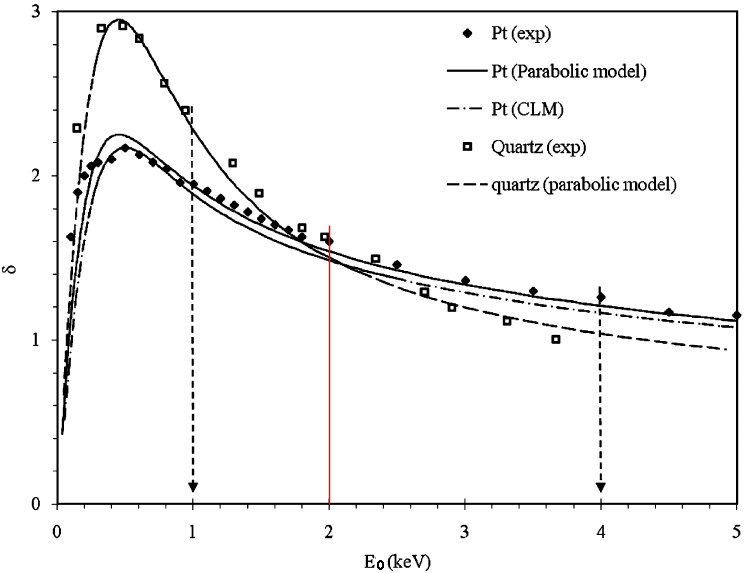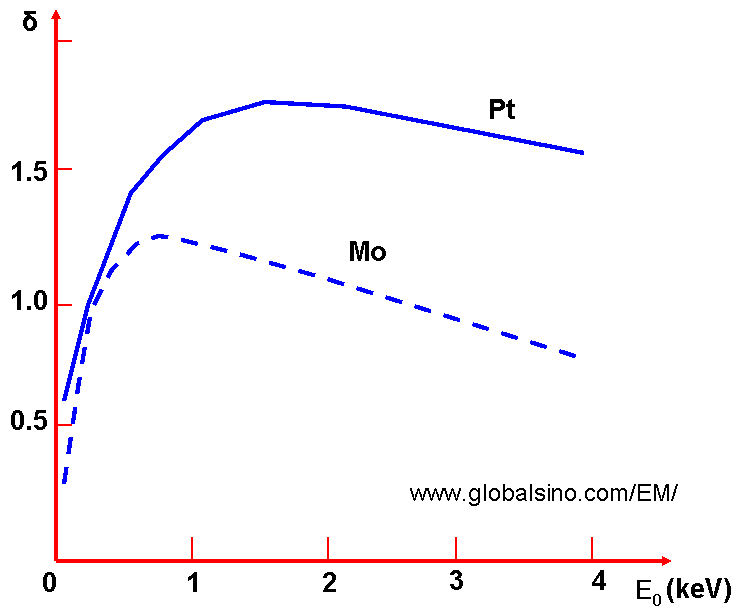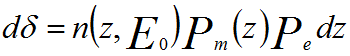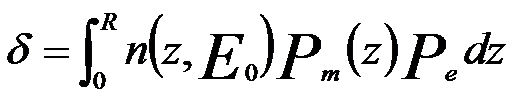Secondary Electron Yield (Emission Coefficient) in SEM
- Practical Electron Microscopy and Database -
- An Online Book -

http://www.globalsino.com/EM/

 This book (Practical Electron Microscopy and Database) is a reference for TEM and SEM students, operators, engineers, technicians, managers, and researchers. ================================================================================= Secondary electron emission (SEE) is a common phenomenon induced by high energy electrons interacting with a specimen. Because the specimen is very thick in SEM observation, almost each incident electron can produce several secondary electrons (SEs). The coefficient of emission, or called as yield, of secondary electrons can be given by δ = iδ/ii. Here, iδ is the total emission current and ii is the current of incident electron beam. It means that the SE yield (δ) is the ratio between the SE current and the primary electron current. In other words, SE yield (δ) can be defined as the number of secondary electrons emitted per incident electron and can be either less than or greater than one. δ depends on the energy (E0) of incident electron beam, angle of incident electron beam, chemical composition, and state of the irradiated sample’s surface. In many cases, the conduction-electron density in metals is high, the SEs have a low probability of escaping the surface of materials; while the concentration of conduction electrons in dielectrics is low, the probability of the escape of SEs is greater. The relative yields of SEs between different materials can change depending on energy of incident electron beam. Figure 4835a shows experimental and theoretical secondary electron yields from quartz  and platinum . The theoretical modeling was performed by constant loss model [3,4] and parabolic model . In the modeling, the researchers considered some factors such as magnitude for SE attenuation length, s, and SE escape probability, A, of the materials of interest. It is very interesting that the crossing of yield curves, δ(E0), is at around E0 = 2 keV. This crossing suggests a contrast change as well as a contrast reversal in the range of incident beam energy from ~1 to ~4 keV as shown by the arrows in the figure.Figure 4835a. Secondary yield curves δf(E0) of Pt and quartz. “exp” represents experimental data. The other ones are simulated data. It is well-known that the probability of secondary electron escape also depends on the height of the potential barrier on the sample surface. As a result, in a number of nonmetallic substances (oxides of alkaline-earth metals; alkali-halide compounds), their yield is much higher than other materials, e.g. as shown in Figure 4835b.Figure 4835b. Dependence of the coefficient of secondary electron emission of NaCl and SiO2 on the energy of the incident electron beam. In Figures 4835a - 4835c, we can notice that σ at first increases with an increase of the energy E0 of the incident electron beam, which takes place until the excitation of the electrons of the solid occurs near the surface at a distance of their path length. Upon further increase of E0, the total number of excited electrons continues to increase, but most of them originate at a greater depth, and the number of SEs that escape decreases.Figure 4835c. Dependence of the coefficient of secondary electron emission of Pt and Mo on the energy of the incident electron beam. Furthermore, many other factors can also enhance SE emission. For instance, SE emission can be enhanced by electrostatic fields and ultrahigh-frequency electrical fields as well as the porosity of materials. In general, the energy dependence on incident electrons shows a maximum δm = 0.35 – 1.6 for metals and 1 – 10 for insulators at energies of Em = 100 – 800 eV and 300 – 2000 eV, respectively.  Here, Em is the energy of incident electrons at which the maximum δm locates. Physically, the SE yield (δ) can be given by,------------- [4835a] where n(z, E0)dz -- Number of SE generated by incident energy E0 in the layer of thickness dz at a depth z below the surface           λ -- Mean free path (MFP)           Pe -- Escape probability (= B) Migration probability to the surface from a depth z is given by,                  Pm(z) = e –z/k ------------------------------------------- [4835b] Constant-loss approximation [4, 7, 8] suggested that the energy dissipation of primary electrons (PE) within the material is approximately constant, the number of primary electrons would decrease linearly to a depth R. The number of SEs produced per unit path length would be a constant, given by,-------- [4835c] where ξ -- Energy required to excite one SE inside the solid           R -- Maximum range of the PE. Combining Equations 4835a and 4835c, we can obtain the yield equation, given by,------------- [4835d] This equation represents integral from the surface (z = 0) to the primary electron range (z = R) as shown in Figure 4835d.Figure 4835d. Schematic showing the maximum primary electron ranges RH, RM, and RL with high, medium, and low electron beam voltages, respectively, for an Al thin film on Si wafer substrate specimen. To know more about the dependence of the emission coefficient of secondary electrons on atomic number and accelerating voltage of incident electron beam, You need to refer to a discussion elsewhere. Furthermore, when the observing structure is electrically isolated, it can be charged during electron irradiation. When the charging process reaches an equilibrium state, the surface potential will maintain constant. In the case of positive charging, the surface potential VS is positive a few volts, retarding the part of the SEs at low-energies and resulting in a lower SE yield. On the other hand, in the case of negative charging, the negative surface potential slightly retards the incident primary electrons, while it increases the SE yield.    H. Salow, Zeit. Fuer Techni. Physik 21 (1940) 1.  C.A.F. Pintȃo, R. Hessel, J. Appl. Phys. 88 (2000) 478.  H. Seiler, J. Appl. Phys. 54 (1983) R1.  D.C. Joy, J. Microsc. 147 (1987) 51.  J. Cazaux, J. Phys. D: Appl. Phys. 38 (2005) 2433.  H. Seiler, J. Appl. Phys. 54 (1983) R1.  Dionne GF (1973) J Appl Phys 44(12):5361  Lye RG, Dekker AJ (1957) Phys Rev 107(4):977

=================================================================================

The book author (Yougui Liao) welcomes your comments, suggestions, and corrections, please click here for submission. If you let book author know once you have cited this book, the brief information of your publication will appear on the “Times Cited” page.